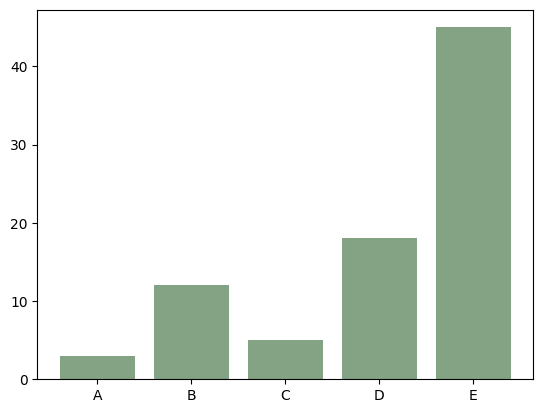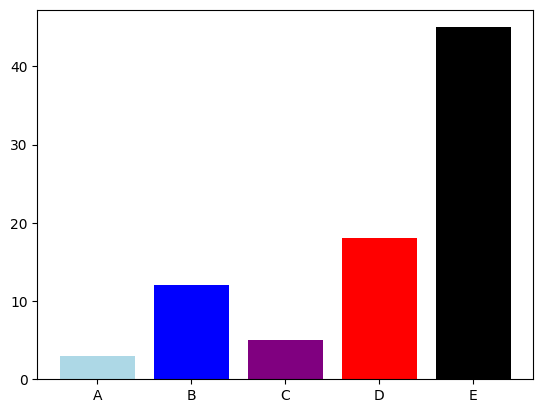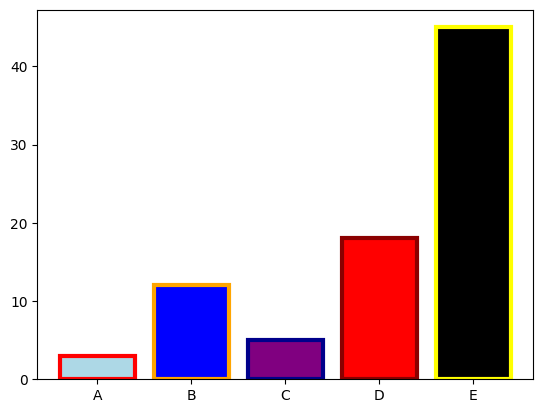## Libraries

First, you need to import the following libraries:

• matplotlib for creating the chart and customize it
• pandas for storing the data used
``````import matplotlib.pyplot as plt
import pandas as pd``````

## Dataset

In this post, we'll use a very simple dataset, that you can create as follow

``````height = [3, 12, 5, 18, 45]
bars = ('A', 'B', 'C', 'D', 'E')

df = pd.DataFrame({'height': height,
'bars': bars})``````

## Uniform color using RGB

You can change the color of bars in a barplot using the `color` argument.
RGB is a way of making colors. You have to to provide an amount of red, green, blue, and the transparency value to the color argument and it returns a color.

``````color = (0.2, # redness
0.4, # greenness
0.2, # blueness
0.6 # transparency
)

# Create bars
fig, ax = plt.subplots()
ax.bar(df['bars'], df['height'],
color=color)

# Show graph
plt.show()``````## Different color for each bar

If you want to give different color to each bar, just provide a list of color names to the `color` argument.

You can find the available colors at this address

``````color = ['lightblue', 'blue', 'purple', 'red', 'black']

# Create bars
fig, ax = plt.subplots()
ax.bar(df['bars'], df['height'],
color=color)

# Show graph
plt.show()``````## Control color of border

The `edgecolor` argument allows you to color the borders of barplots. You can either put a color name like 'blue' or put a list of colors.

We also specify `linewidth=3` in order to make the edges more visible (the default value is 1 and can be too thin).

``````color = ['lightblue', 'blue', 'purple', 'red', 'black']
edgecolor = ['red', 'orange', 'darkblue', 'darkred', 'yellow']

# Create bars
fig, ax = plt.subplots()
ax.bar(df['bars'], df['height'],
color=color,
edgecolor=edgecolor,
linewidth=3)

# Show graph
plt.show()``````## Going further

This post explains how to modify the colors of a barplot with matplotlib.

You might want to check how to custom width of bars and how to flip a barplot.

## Contact & Edit

👋 This document is a work by Yan Holtz. You can contribute on github, send me a feedback on twitter or subscribe to the newsletter to know when new examples are published! 🔥